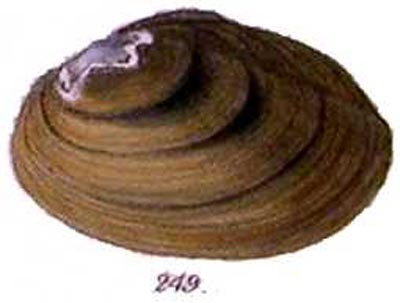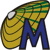MUSSELpdb Introduction Browse d'basing

Page last updated
9 August
2018

Click here to read the database disclaimer.

# The Freshwater Mussels (Unionoida) of the World(and other less consequential bivalves)

FM(U)otW(aolcb) is the web version of the MUSSEL Project Database. Follow the links to browse the data or use the search fields. Either way, you win!

Taxa known only as fossils are highlighted in gray. Those without representatives in fresh waters are highlighted in gold.

 Search: scientific namenominal speciesvalid genusnominal genusspecimen locality

 Bivalvia top
 NOTICE! This version of the MUSSELpdb online is no longer supported or up-to-date. It will be removed from this web site in the summer of 2019.Click here to view these data in the current, updated version. Or, click here to browse from the starting page.

Publication Information

Lydeard, C., J.T. Garner, P. Hartfield & J.D. Williams. 1999. Freshwater mussels in the Gulf Region: Alabama. Gulf of Mexico Science 17(2): 125-134.

##### Species Described
Unio hanleyanus Conrad, 1853.
 ‘Lea’ Of Authors. Conrad, 1853, Proc. Acad. Nat. Sci.: 250. Sowerby, 1866, Conch. Icon.: pl. 46, sp. 249. Simpson, 1900, Proc. U.S. Nat. Mus.: 759. Simpson, 1914, Descr. Cat. Naiades: 780. Frierson, 1927, Check List N. Amer. Naiades: 42, sp. 175. Haas, 1969, Das Tierreich: 256. Burch, 1975, Freshw. Unio. North Amer.: 13. Turgeon et al., 1988, Am. Fisher. Soc. Spec. Publ.: 32. Williams et al., 1993, Fisheries: 13. Parmalee & Bogan, 1998, The Freshwater Mussels of Tennessee: 184. Lydeard et al., 1999, Gulf Mex. Sci.: 129.
(error for Unio hanleyianus Lea, 1852)
= Pleurobema hanleyianum##### Taxonomic Opinions
Actinonaias ligamentina (Lamarck). — Lydeard et al. (1999: 126).
Actinonaias pectorosa (Conrad). — Lydeard et al. (1999: 126).
Alasmidonta marginata Say. — Lydeard et al. (1999: 126).
Alasmidonta mccordi Athearn. — Lydeard et al. (1999: 126).
Alasmidonta triangulata (Say) [sic]. — Lydeard et al. (1999: 126).
Alasmidonta viridis (Rafinesque). — Lydeard et al. (1999: 126).
Amblema elliottii (Lea). — Lydeard et al. (1999: 126).
Amblema plicata (Conrad) [sic]. — Lydeard et al. (1999: 126).
Anodonta suborbiculata Say. — Lydeard et al. (1999: 126).
Anodontoides radiatus (Conrad). — Lydeard et al. (1999: 126).
Arcidens confragosus (Say). — Lydeard et al. (1999: 126).
Cumberlandia monodonta (Say). — Lydeard et al. (1999: 126).
Cyclonaias tuberculata (Rafinesque). — Lydeard et al. (1999: 126).
Cyprogenia stegaria (Rafinesque). — Lydeard et al. (1999: 126).
Dromus dromas (Lea). — Lydeard et al. (1999: 126).
Ellipsaria lineolata (Rafinesque). — Lydeard et al. (1999: 126).
Elliptio arca (Conrad). — Lydeard et al. (1999: 126).
Elliptio arctata (Conrad). — Lydeard et al. (1999: 126).
Elliptio chipolaensis Walker. — Lydeard et al. (1999: 126).
Elliptio complanata (Lightfoot). — Lydeard et al. (1999: 126).
Elliptio crassidens (Lamarck). — Lydeard et al. (1999: 126).
Elliptio dilatata (Rafinesque). — Lydeard et al. (1999: 126).
Elliptio fraterna (Lea). — Lydeard et al. (1999: 126).
Elliptio icterina (Conrad). — Lydeard et al. (1999: 126).
Elliptio mcmichaeli Clench & Turner. — Lydeard et al. (1999: 126).
Elliptio nigella (Lea). — Lydeard et al. (1999: 126).
Elliptoideus sloatianus (Lea). — Lydeard et al. (1999: 126).
Epioblasma arcaeformis (Lea). — Lydeard et al. (1999: 126).
Epioblasma biemarginata (Lea). — Lydeard et al. (1999: 126).
Epioblasma brevidens (Lea). — Lydeard et al. (1999: 126).
Epioblasma capsaeformis (Lea). — Lydeard et al. (1999: 127).
Epioblasma flexuosa (Rafinesque). — Lydeard et al. (1999: 127).
Epioblasma florentina florentina (Lea). — Lydeard et al. (1999: 127).
Epioblasma haysiana (Lea). — Lydeard et al. (1999: 127).
Epioblasma lenior (Lea). — Lydeard et al. (1999: 127).
Epioblasma lewisii (Walker). — Lydeard et al. (1999: 127).
Epioblasma metastriata (Conrad). — Lydeard et al. (1999: 127).
Epioblasma obliquata obliquata (Rafinesque). — Lydeard et al. (1999: 127).
Epioblasma othcaloogensis (Lea). — Lydeard et al. (1999: 127).
Epioblasma penita (Conrad). — Lydeard et al. (1999: 127).
Epioblasma personata (Say). — Lydeard et al. (1999: 127).
Epioblasma propinqua (Lea). — Lydeard et al. (1999: 127).
Epioblasma stewardsonii (Lea). — Lydeard et al. (1999: 127).
Epioblasma torulosa torulosa (Rafinesque). — Lydeard et al. (1999: 127).
Epioblasma triquetra (Rafinesque). — Lydeard et al. (1999: 127).
Epioblasma turgidula (Lea). — Lydeard et al. (1999: 127).
Fusconaia barnesiana (Lea). — Lydeard et al. (1999: 127).
Fusconaia cerina (Conrad). — Lydeard et al. (1999: 127).
Fusconaia cor (Conrad). — Lydeard et al. (1999: 127).
Fusconaia cuneola (Lea). — Lydeard et al. (1999: 127).
Fusconaia ebena (Lea). — Lydeard et al. (1999: 127).
Fusconaia escambia Clench & Turner. — Lydeard et al. (1999: 127).
Fusconaia subrotunda (Lea). — Lydeard et al. (1999: 127).
Fusconaia succissa (Lea). — Lydeard et al. (1999: 127).
Glebula rotundata (Lamarck). — Lydeard et al. (1999: 127).
Hemistena lata (Rafinesque). — Lydeard et al. (1999: 127).
Lampsilis abrupta (Say). — Lydeard et al. (1999: 127).
Lampsilis altilis (Conrad). — Lydeard et al. (1999: 127).
Lampsilis australis Simpson. — Lydeard et al. (1999: 127).
Lampsilis binominata Simpson. — Lydeard et al. (1999: 127).
Lampsilis cardium Rafinesque. — Lydeard et al. (1999: 127).
Lampsilis fasciola Rafinesque. — Lydeard et al. (1999: 127).
Lampsilis haddletoni Athearn. — Lydeard et al. (1999: 127).
Lampsilis ornata (Conrad). — Lydeard et al. (1999: 127).
Lampsilis ovata (Say). — Lydeard et al. (1999: 128).
Lampsilis perovalis (Conrad). — Lydeard et al. (1999: 128).
Lampsilis straminea claibornensis (Lea). — Lydeard et al. (1999: 128).
Lampsilis straminea straminea (Conrad). — Lydeard et al. (1999: 128).
Lampsilis subangulata (Lea). — Lydeard et al. (1999: 128).
Lampsilis teres (Rafinesque). — Lydeard et al. (1999: 128).
Lampsilis virescens (Lea). — Lydeard et al. (1999: 128).
Lasmigona complanata alabamensis Clarke. — Lydeard et al. (1999: 128).
Lasmigona complanata complanata (Barnes). — Lydeard et al. (1999: 128).
Lasmigona costata (Rafinesque). — Lydeard et al. (1999: 128).
Lasmigona holstonia (Lea). — Lydeard et al. (1999: 128).
Lasmigona subviridis (Conrad). — Lydeard et al. (1999: 128).
Lemiox rimosus (Rafinesque). — Lydeard et al. (1999: 128).
Leptodea fragilis (Rafinesque). — Lydeard et al. (1999: 128).
Leptodea leptodon (Rafinesque). — Lydeard et al. (1999: 128).
Lexingtonia dolabelloides (Lea). — Lydeard et al. (1999: 128).
Ligumia recta (Lamarck). — Lydeard et al. (1999: 128).
Ligumia subrostrata (Say). — Lydeard et al. (1999: 128).
Margaritifera marrianae R.I. Johnson. — Lydeard et al. (1999: 126).
Medionidus acutissimus (Lea). — Lydeard et al. (1999: 128).
Medionidus conradicus (Lea). — Lydeard et al. (1999: 128).
Medionidus mcglameriae van der Schalie. — Lydeard et al. (1999: 128).
Medionidus parvulus (Lea). — Lydeard et al. (1999: 128).
Medionidus penicillatus (Lea). — Lydeard et al. (1999: 128).
Megalonaias nervosa (Rafinesque). — Lydeard et al. (1999: 128).
Obliquaria reflexa Rafinesque. — Lydeard et al. (1999: 128).
Obovaria jacksoniana (Frierson). — Lydeard et al. (1999: 128).
Obovaria olivaria (Rafinesque). — Lydeard et al. (1999: 128).
Obovaria retusa (Lamarck). — Lydeard et al. (1999: 128).
cf. Obovaria rotulata (Wright). — Lydeard et al. (1999: 128).
Obovaria subrotunda (Rafinesque). — Lydeard et al. (1999: 128).
Obovaria unicolor (Lea). — Lydeard et al. (1999: 128).
Pegias fabula (Lea). — Lydeard et al. (1999: 128).
Plectomerus dombeyanus (Valenciennes). — Lydeard et al. (1999: 128).
Plethobasus cicatricosus (Say). — Lydeard et al. (1999: 128).
Plethobasus cooperianus (Lea). — Lydeard et al. (1999: 129).
Plethobasus cyphyus (Rafinesque). — Lydeard et al. (1999: 129).
Pleurobema altum (Conrad). — Lydeard et al. (1999: 129).
Pleurobema avellanum Simpson. — Lydeard et al. (1999: 129).
Pleurobema chattanoogaense (Lea). — Lydeard et al. (1999: 129).
Pleurobema clava (Lamarck). — Lydeard et al. (1999: 129).
Pleurobema cordatum (Rafinesque). — Lydeard et al. (1999: 129).
Pleurobema curtum (Lea). — Lydeard et al. (1999: 129).
Pleurobema decisum (Lea). — Lydeard et al. (1999: 129).
Pleurobema flavidulum (Lea). — Lydeard et al. (1999: 129).
Pleurobema furvum (Conrad). — Lydeard et al. (1999: 129).
Pleurobema georgianum (Lea). — Lydeard et al. (1999: 129).
Pleurobema hagleri (Frierson). — Lydeard et al. (1999: 129).
Pleurobema hanleyanum [sic] (Lea). — Lydeard et al. (1999: 129).
Pleurobema johannis (Lea). — Lydeard et al. (1999: 129).
Pleurobema marshalli Frierson. — Lydeard et al. (1999: 129).
Pleurobema murrayense (Lea). — Lydeard et al. (1999: 129).
Pleurobema nucleopsis (Conrad). — Lydeard et al. (1999: 129).
Pleurobema oviforme (Conrad). — Lydeard et al. (1999: 129).
Pleurobema perovatum (Conrad). — Lydeard et al. (1999: 129).
Pleurobema plenum (Lea). — Lydeard et al. (1999: 129).
Pleurobema pyriforme (Lea). — Lydeard et al. (1999: 129).
Pleurobema rubellum (Conrad). — Lydeard et al. (1999: 129).
Pleurobema rubrum (Rafinesque). — Lydeard et al. (1999: 129).
Pleurobema sintoxia (Rafinesque). — Lydeard et al. (1999: 129).
Pleurobema strodeanum (Wright). — Lydeard et al. (1999: 129).
Pleurobema taitianum (Lea). — Lydeard et al. (1999: 129).
Pleurobema troschelianum (Lea). — Lydeard et al. (1999: 129).
Pleurobema verum (Lea). — Lydeard et al. (1999: 129).
Potamilus alatus (Say). — Lydeard et al. (1999: 129).
Potamilus inflatus (Lea). — Lydeard et al. (1999: 129).
Potamilus ohiensis (Rafinesque). — Lydeard et al. (1999: 129).
Potamilus purpuratus (Lamarck). — Lydeard et al. (1999: 129).
Ptychobranchus fasciolaris (Rafinesque). — Lydeard et al. (1999: 129).
Ptychobranchus greenii (Conrad). — Lydeard et al. (1999: 130).
Ptychobranchus jonesi (van der Schalie). — Lydeard et al. (1999: 130).
Ptychobranchus subtentum (Say). — Lydeard et al. (1999: 130).
Pyganodon cataracta (Say). — Lydeard et al. (1999: 130).
Pyganodon grandis (Say). — Lydeard et al. (1999: 130).
Quadrula apiculata (Say). — Lydeard et al. (1999: 130).
Quadrula asperata (Lea). — Lydeard et al. (1999: 130).
Quadrula cylindrica cylindrica (Say). — Lydeard et al. (1999: 130).
Quadrula fragosa (Conrad). — Lydeard et al. (1999: 130).
Quadrula intermedia (Conrad). — Lydeard et al. (1999: 130).
Quadrula metanevra (Rafinesque). — Lydeard et al. (1999: 130).
Quadrula nodulata (Rafinesque). — Lydeard et al. (1999: 130).
Quadrula pustulosa pustulosa (Lea). — Lydeard et al. (1999: 130).
Quadrula quadrula (Rafinesque). — Lydeard et al. (1999: 130).
Quadrula rumphiana (Lea). — Lydeard et al. (1999: 130).
Quadrula stapes (Lea). — Lydeard et al. (1999: 130).
Quincuncina burkei Walker. — Lydeard et al. (1999: 130).
Quincuncina infucata (Conrad). — Lydeard et al. (1999: 130).
Strophitus connasaugaensis (Lea). — Lydeard et al. (1999: 130).
Strophitus subvexus (Conrad). — Lydeard et al. (1999: 130).
Strophitus undulata [sic] (Say). — Lydeard et al. (1999: 130).
Toxolasma corvunculus (Lea). — Lydeard et al. (1999: 130).
Toxolasma cylindrellus (Lea). — Lydeard et al. (1999: 130).
Toxolasma lividus (Rafinesque). — Lydeard et al. (1999: 130).
Toxolasma parvus (Barnes). — Lydeard et al. (1999: 130).
Toxolasma paulus (Lea). — Lydeard et al. (1999: 130).
Tritogonia verrucosa (Rafinesque). — Lydeard et al. (1999: 130).
Truncilla donaciformis (Lea). — Lydeard et al. (1999: 130).
Truncilla truncata Rafinesque. — Lydeard et al. (1999: 130).
Uniomerus caroliniana (Bosc). — Lydeard et al. (1999: 130).
Uniomerus declivis (Say). — Lydeard et al. (1999: 130).
Uniomerus tetralasmus (Say). — Lydeard et al. (1999: 130).
Utterbackia imbecillis (Say). — Lydeard et al. (1999: 130).
Utterbackia peggyae (Johnson). — Lydeard et al. (1999: 130).
Villosa choctawensis Athearn. — Lydeard et al. (1999: 131).
Villosa fabalis (Lea). — Lydeard et al. (1999: 131).
Villosa iris (Lea). — Lydeard et al. (1999: 131).
Villosa lienosa (Conrad). — Lydeard et al. (1999: 131).
Villosa nebulosa (Conrad). — Lydeard et al. (1999: 131).
Villosa taeniata (Conrad). — Lydeard et al. (1999: 131).
Villosa trabalis (Conrad). — Lydeard et al. (1999: 131).
Villosa vanuxemensis umbrans (Lea). — Lydeard et al. (1999: 131).
Villosa vanuxemensis vanuxemensis (Lea). — Lydeard et al. (1999: 131).
Villosa vibex (Conrad). — Lydeard et al. (1999: 131).